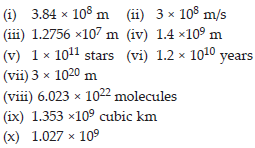Exponents and Power (Maths) Class 7 - NCERT Questions
Q 1.

Find the value of:
(i) 26 (ii) 93
(iii) 112 (iv) 54

SOLUTION: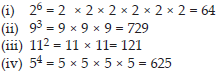Q 2.

Express the following in exponential form:
(i) 6 × 6 × 6 × 6 (ii) t × t
(iii) b × b × b × b (iv) 5 × 5× 7 × 7 × 7
(v) 2 × 2 × a × a
(vi) a × a × a × c × c × c × c × d

SOLUTION: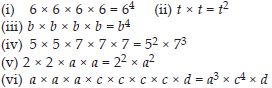Q 3.

Express each of the following numbers using exponential notation:
(i) 512 (ii) 343
(iii) 729 (iv) 3125

SOLUTION: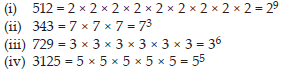Q 4.

Identify the greater number, wherever possible, in each of the following?
(i) 43 or 34 (ii) 53 or 35
(iii) 28 or 82 (iv) 1002 or 2100
(v) 210 or 1002

SOLUTION: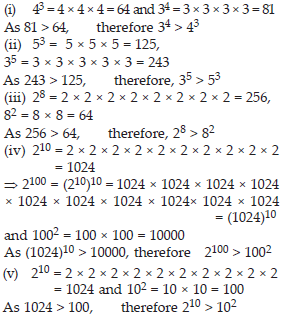Q 5.

Express each of the following as product of powers of their prime factors:
(i) 648 (ii) 405
(iii) 540 (iv) 3600

SOLUTION: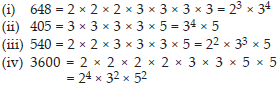Q 6.

Simplify:
(i) 2 × 103 (ii) 72 × 22
(iii) 23 × 5 (iv) 3 × 44
(v) 0 × 102 (vi) 52 × 33
(vii) 24 × 32 (viii) 32 × 104

SOLUTION: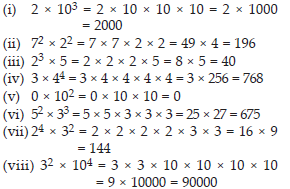Q 7.

Simplify:
(i) (– 4)3 (ii) (–3) × (–2)3
(iii) (–3)2 × (–5)2 (iv) (–2)3 × (–10)3

SOLUTION: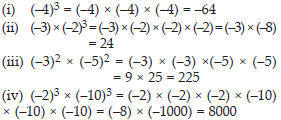Q 8.

Compare the following numbers:
(i) 2.7 × 1012 ; 1.5 × 108
(ii) 4 × 1014 ; 3 × 1017)

SOLUTION: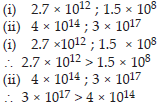Q 9.

Using laws of exponents, simplify and write the answer in exponential form: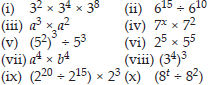SOLUTION: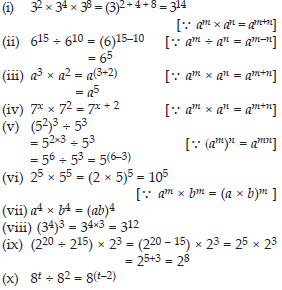Q 10.

Simplify and express each of the following in exponential form: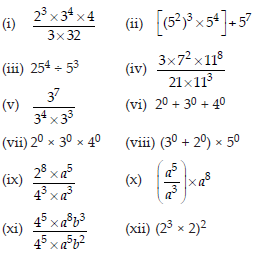SOLUTION: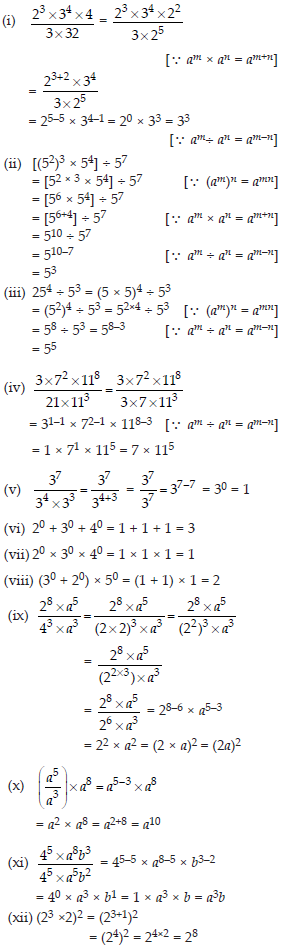Q 11.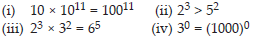SOLUTION: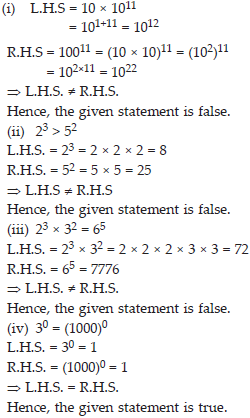Q 12.

Express each of the following as a product of prime factors only in exponential form:
(i) 108 ×192 (ii) 270
(iii) 729 × 64 (iv) 768

SOLUTION: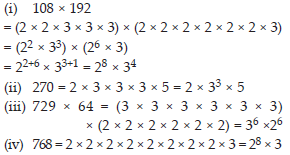Q 13.

Simplify: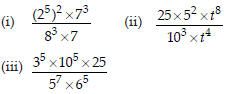SOLUTION: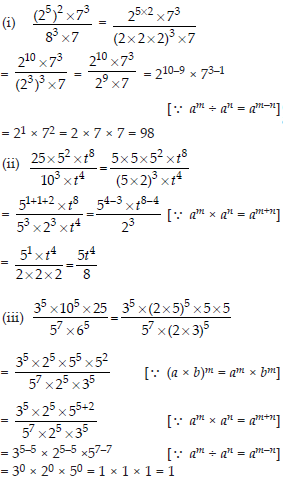Q 14.

Write the following numbers in the expanded forms:
279404, 3006194, 2806196, 120719, 20068

SOLUTION: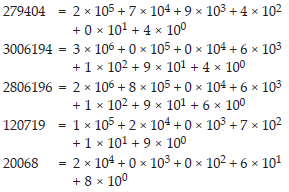Q 15.

Find the number from each of the following expanded forms: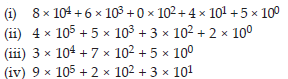SOLUTION: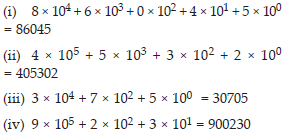Q 16.

Express the following numbers in standard form:
(i) 5,00,00, 000 (ii) 70, 00, 000
(iii) 3, 18, 65, 00, 000
(iv) 3, 90, 878
(v) 39087.8 (vi) 3908.78

SOLUTION: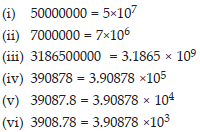Q 17.

Express the number appearing in the following statements in standard form.
(i) The distance between Earth and Moon is 384, 000, 000 m.
(ii) S Speed of light in vacuum is 300,000,000 m/s
(iii) Diameter of the Earth is 1,27,56,000 m.
(iv) Diameter of the Sun is 1,400,000,000 m.
(v) In a galaxy there are on an average 100,000,000,000 stars.
(vi) The universe is estimated to be about 12, 000, 000, 000 years old.
(vii) The distance of the Sun from the centre of the Milky Way Galaxy is estimated to be 300, 000, 000, 000, 000, 000, 000 m.
(viii) 60, 230, 000, 000, 000, 000, 000, 000 molecules are contained in a drop of water weighing 1.8 gm
(ix) The earth has 1, 353, 000, 000 cubic km of sea water.
(x) The population of India was about 1, 027, 000, 000 in March, 2001.

SOLUTION: